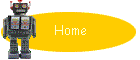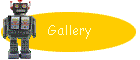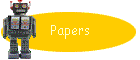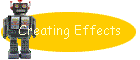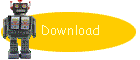Pan is embedded in the functional programming language Haskell. See the Haskell home page for a wealth of info about Haskell.  In particular, check out various bits of documentation on Hugs98, A Gentle Introduction to Haskell, and the Haskell language and library definitions for full details.  In this section, we present just enough Haskell to get working with Pan.

## Definitions

A Haskell program is made up of a collection of definitions. For instance,
 ```msg = "Shall we get started?" msgSize = length msg```

The first two lines define the name msg to be a particular string, size to be the length of (number of characters in) msg. Note in the second line that application of a function (length) to an argument (msg) is written simply by juxtaposing the function and argument, without requiring parentheses around the argument.

A function definition is written similarly, but with the formal parameter name(s) given on the left hand side, as in the following definition of greeting, which uses the infix concatenation operator, "++".
 ``` greeting name = "Hello, " ++ name ++ ". " ++ msg ```

## Types

Haskell is statically typed, but in most cases, the compiler or interpreter can infer all types automatically. For the three names defined above, the following declarations may be either stated by the programmer or left to be inferred:
 ```msg :: String msgSize :: Int greeting :: String -> String ```
The last declaration means "function from String to String". Note that, in Haskell, type names begin with capital letters, while value and function names begin with lowers letters.

## Multiple arguments

Application and definition of functions with more than one argument are written using repeated juxtaposition. For instance, the following defines a function strangeName of two arguments, making use of the function elem of two arguments. It considers a name to be strange if the name contains a 'z' and is longer than a given length.
 ```strangeName name n = (elem 'z' name) && (length name > n)```

The types of elem (a Haskell standard library function) and strangeName could be
 ```strangeName :: String -> Int -> Bool elem :: Char -> String -> Bool ```
where Bool is the type of Booleans, i.e., true/false values.

The function type operator, "->", is right associative, so this declaration literally says that elem takes a character and returns a function that takes a string and returns a boolean. Correspondingly, function application is left associative, so the definition above applies elem to 'z', returning a function that is applied to name.  The notation may seem odd at first, but has a very useful property: functions may be partially applied, to synthesize a function of fewer arguments.  For example:
 ```hasZ :: String -> Bool hasZ = elem 'z' haveZ :: String -> String -> Bool haveZ str1 str2 = hasZ str1 && hasZ str2 ```

The trick of turning n-ary functions into function-producing functions is known as "Currying" in honor of Haskell Curry.  (Yes, the Haskell language is also named after him.)

## Polymorphism

It is not really satisfactory for the elem function to have the type given above. In fact, it applies not just to strings, i.e., sequences of characters, but to sequences of any type of values. The type name "String" is just an abbreviation for list of characters
 `type String = [Char]`
and real type of elem is the following:
 `elem :: a -> [a] -> Bool`

In type declarations, the use of a non-capitalized name indicates a type variable. This declaration says that the first argument to elem has some type a, and the second is a list of values, each having the same type a. (Note that the substitution for a is uniform. The sought element and every member of the list must all have the same type.) In a definition like that of strangeName above, the compiler figures out how to specialize the type of elem to the context of its use, in this case on characters.

Note that there is not a single "list" type, but rather a list "type constructor" or, in C++ terms, a "template type", parameterized over arbitrary element types. One may define additional type constructors as well. For instance, the notion of binary trees with values at the leaves might be captured by a type constructor BinTree, so a tree of strings would have type BinTree String. Type constructors may have any number of type arguments.  Since lists are so useful, Haskell provides the syntax "[a]" rather than "List a".  Other generally useful type constructors include "->" (binary) and "(,...,)" (binary, ternary, etc) for forming function and tuple types respectively.

## Infix operators

Note that the operators "&&" and ">" are used as infix. Such operators are simply function names whose names are composed of nonalphanumeric characters. By default, these names must be used as infix. Alphanumeric names precede their arguments, but may be used in infix form if surrounded by backquotes, as in the following expression.
 `'z' `elem` name`

Names may also have declared syntactic binding strengths (precedences), to reduce the need for parentheses. All infix applications bind less strongly than function application (juxtaposition), so the parentheses is the definition of strangeName are unnecessary (because "&&" binds less tightly than ">").

The operator "\$" is an infix version of function application.  It's often used to eliminate need for parentheses, which helps readability (in some eyes).  You can find its definition in lib/Prelude.hs in your Hugs installation.
 ```infixr 0 \$ (\$) :: (a -> b) -> a -> b f \$ x = f x```

The first line says that "\$" is infix, right-associative, and of lowest syntactic precedence.

For instance, suppose we want to map the functions h, g, and f over a list l.  We could say
 `map f (map g (map h l)))`
or
 `map f \$ map g \$ map h l`

Another useful operator is function composition, notated ".".  A semantically equivalent version of the f, g, h example is
 `map (f . g . h) l`

## Local definitions

It is often convenient to introduce some definitions just in order to make another definition more efficient or readable. In such a case, one may introduce local definitions, prefixed by "where".  For instance:
 ```thriceLength name = n + m where n = length name m = n + n```

Here, the scope of the names n and m include the right hand side the definition of thriceLength, as well as the right hand side the definitions of n and m themselves. Thus order does not matter (and the definitions are mutually recursive, as are definitions at top level scope).

Functions may be defined locally as well as non-functions.

## Anonymous functions

Partial application of functions creates new functions and is a simple technique if you can come up arguments for the first one or more arguments.  Local definition of functions is a somewhat more general technique of function construction.  A third notational technique is to use "lambda notation" for anonymous functions.  The expression " \ pattern -> body " means a function that matches its argument against pattern and returns the value of body.  Usually patterns are simply a variable or tuple of variables, but they may include literals and nested tuples.

As an example of anonymous functions at work, the following expression maps a function over the list [1,2,...,10].  The mapped function takes an argument x, and returns four more than thrice x.
 `map (\x -> 3 * x + 4) [1 .. 10]`

## Indentation

Unlike most programming languages, indentation matters in Haskell.  The details are complicated, but in practice you can use reasonable indentation practice and all works out well.  The benefit is to eliminate most much explicit punctuation or bracketing.